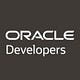Linear Regression — Machine Learning with TensorFlow and Oracle JET UI Explained

Machine learning topic is definitely popular these days. Some get wrong assumptions about it — they think machine could learn by itself and its kind of magic. The truth is — there is no magic, but math behind it. Machine will learn the way math model is defined for learning process. In my opinion, the best solution is a combination of machine learning math and algorithms. Here I could relate to chatbots keeping conversational context — language processing can be done by machine learning with neural network, while intent and context processing can be executed by programmable algorithms.

If you are starting to learn machine learning — there are two essential concepts to start with:

1. Regression

2. Classification

This post is focused around regression, in the next posts I will talk about classification.

Regression is a method which calculates the best fit for a curve to summarize data. Its up to you which type of curve to choose, you should assume which type will be most suitable (this can be achieved with trial and error too) based on given data set. Regression goal is to understand data points by discovering the curve that might have generated them.

In this example I will be using simplest regression possible — linear. Line is described by equation y = W*x + b. Where b is optional and can be 0 (line will cross (0, 0) point). For complex data sets, we might use polynomial equations and generate curves.

Here is Python code which implements linear regression with TensorFlow API (I have provided comments for all steps, reading code should be self explanatory):

Key element in any kind of machine learning — cost. The higher the cost, the worse is learning output. In linear regression, cost is typically defined by the sum of errors. The error in predicting x is calculated by the squared difference between the actual value f(x) and the predicted value M(w, x). The cost is the sum of squared differences between the actual and predicted values.

As you can see in the code above, we define cost function and ask TensorFlow to run optimizer to find the optimal values for model parameters. All the hard math calculation is happening in TensorFlow, our job is to prepare training data and choose right learning approach with correct equation.

Let’s run JET UI, which talks to TensorFlow through REST. Training data is randomly generated (always 100 points) during each training session.

Training Epochs — number of learning iterations during training session Learning rate — learning speed, smaller rate — more careful learning
W — learned model parameter to calculate equation y = W*x
Cost — value which shows how successful learning was, lower cost is better

1. We start from 1 training epoch and learning rate 0.001:

Learning result is not good — red line is result of linear regression, it doesn’t represent best fit for training data. Cost is pretty high too, which indicates that learning wasn’t successful.

2. 10 training epochs and learning rate 0.001:

As we repeat multiple learning iterations within the same training session — learning result is better. Cost becomes smaller and linear regression calculated line fits better, but still not ideal.

3. 100 training epochs and learning rate 0.001:

It helps to increase learning iterations, cost is significantly improving and line fits much better. This means outcome for W parameter learning is pretty good.

4. 1000 training epochs and learning rate 0.001

Let’s make model to learn even harder and repeat more times — cost is becoming even better.

5. 2000 training epochs and learning rate 0.001

We could increase learning iterations further, but at some point it will not help. Learning process will start to suffer from overfitting. You can think about it — learning and repeating so many times, that at the end you start forgetting things. Cost is getting worse when repeating learning iterations further.

6. 2000 training epochs and learning rate 0.0001

It should help to make learning rate smaller, which result in more careful learning. This should allow to get better learning results with higher number of learning iterations. We get best learning cost result here and the most optimal line. You may ask — what is the use of that line? It can help to predict y values which were not available in training dataset.

7. 2000 training epochs and learning rate 0.01

On contrary if we increase learning rate, learning process will be faster — optimizer will run faster. This will result in decreased model output quality, cost will be higher and W parameter value will not produce such best fit line as in previous training run.

Few hints related to Oracle JET UI. You can achieve very good data visualization with JET chart components. For example I could control marker type rendered for training data points:

Line which represent learning result, can be displayed as reference line:

To display reference line, I’m using y-axis property which comes with JET chart:

References:

Originally published at andrejusb.blogspot.com on January 16, 2018.

Oracle Developers

A community for developers by developers.

Aggregation of articles from Oracle engineers, Groundbreaker Ambassadors, Oracle ACEs, and Java Champions on all things Oracle technology. The views expressed are those of the authors and not necessarily of Oracle.

Written by

Andrej Baranovskij

TensorFlow Certified Developer | https://github.com/katanamlOracle Developers

Aggregation of articles from Oracle engineers, Groundbreaker Ambassadors, Oracle ACEs, and Java Champions on all things Oracle technology. The views expressed are those of the authors and not necessarily of Oracle.